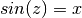# numpy.arcsin¶

numpy.arcsin(x[, out])

Inverse sine, element-wise.

Parameters : x : array_like y-coordinate on the unit circle. out : ndarray, optional Array of the same shape as x, in which to store the results. See doc.ufuncs (Section “Output arguments”) for more details. angle : ndarray The inverse sine of each element in x, in radians and in the closed interval [-pi/2, pi/2]. If x is a scalar, a scalar is returned, otherwise an array.

sin, cos, arccos, tan, arctan, arctan2, emath.arcsin

Notes

arcsin is a multivalued function: for each x there are infinitely many numbers z such that. The convention is to return the angle z whose real part lies in [-pi/2, pi/2].

For real-valued input data types, arcsin always returns real output. For each value that cannot be expressed as a real number or infinity, it yields nan and sets the invalid floating point error flag.

For complex-valued input, arcsin is a complex analytic function that has, by convention, the branch cuts [-inf, -1] and [1, inf] and is continuous from above on the former and from below on the latter.

The inverse sine is also known as asin or sin^{-1}.

References

Abramowitz, M. and Stegun, I. A., Handbook of Mathematical Functions, 10th printing, New York: Dover, 1964, pp. 79ff. http://www.math.sfu.ca/~cbm/aands/

Examples

```>>> np.arcsin(1)     # pi/2
1.5707963267948966
>>> np.arcsin(-1)    # -pi/2
-1.5707963267948966
>>> np.arcsin(0)
0.0
```

numpy.tan

numpy.arccos